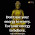Hello,

Today, I am publishing arithmetic maths by devendra sir.
This book is very useful for many competitive exam like ( SSC, CGL, CHSL, CPO, FCI, MTS, IAS, PCS, RAS, RAILWAY, BANK OR OTHER EXAM). This book is in hand written notes format.

CONTENTS

1. NUMBER SYSTEM
2. UNIT DIGITS
3. REMAINDER THEOREM
4. FRACTION & BODMAS RULE
5. DESIMAL FRACTION
6. NUMBER OF FRACTION
7. NUMBER OF ZEROS
8. BINOMIAL THEOREM
9. NUMBER OF DIGITS
10. TYPES OF NUMBERS
11. RULES OF DIVISIBILITY
12. SQUARE & SQUARE ROOTS
13. CUBE & CUBE ROOTS
14. SIMPLIFICATION

ANY SUGGESTIONS CONTACT OR  INFORM US.